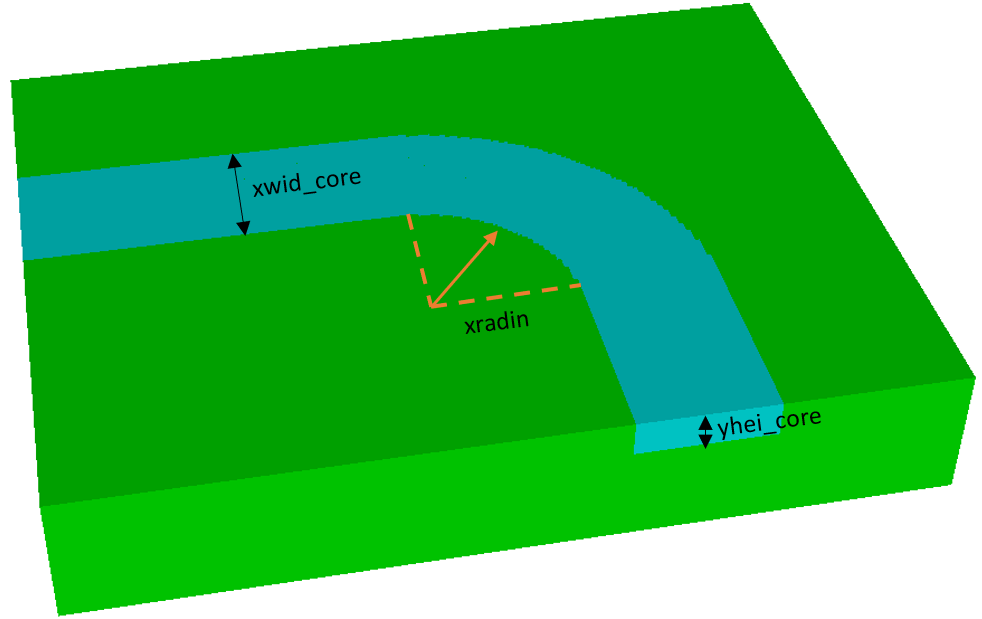# Curved Waveguide Monte Carlo Study

Monte Carlo Simulation a statistical method which uses simulation to model the probability of outcomes of a complex model whose behavior cannot be easily determined due to a vast number of variables.

In this example we vary three parameters of a curved waveguide: core width, core thickness and inner bend radius and analyse the effect on transmission coefficient.

## Model Setup

A schematic of the model and the three input variables can be seen below:The key model parameters were as follows. The variables to be randomly varied are indicated by *

Design Variable Description Default Value
xwid_core * Width of core 1500 nm
yhei_core * Thickness of core 800 nm
yhei_model Total thickness of model 3000 nm

500 random input variables were created with the following constraints:

• Core width: 1500 nm ± 10%
• Core thickness: 800 nm ± 10%
• Inner bend radius: 1700 nm ± 25%

## Monte Carlo Results

The full study was completed in 7 minutes when using 2 cores per simulation and had a total cost of 76.28 Core-Hours.

The transmission coefficient was calculated using the power in and out of the waveguide. To see the affect the four parameters had on transmission coefficient results are plotted below in MATLAB.

The Monte Carlo study shows that there is a spread of transmission coefficients between ~70% and ~97.5% with more being on the higher end of that scale. The study also shows there is a direct correlation between core thickness and transmission as well as inner bend radius and transmission.

## Try this Yourself

To run this Monte Carlo study you will need to download the OnScale and MATLAB files.